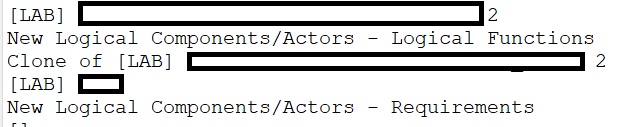# Get_representing_diagrams() VS Semantic Browser Current Element

Hello,
I have been experimenting with (get_representing_diagrams()) on a Logical Component And I noticed there was only a specific type of diagrams returned, other types are missing.

Let’s compare the diagrams returned in P4C and the the diagrams shown in the “Semantic Browser” tab of Capella, for the same Capella Element (A logical Component).

First the script:

• First I got a Python Object of the Logical Component: <main.LogicalComponent object at 0x0000016AFC467108>
• I applied the function Get represeting diagrams and obtained this list of diagrams: [<main.Diagram object at 0x0000016AFBFF8E08>, <main.Diagram object at 0x00…>…]

The names of each diagram is:This is a list of 3 LABS and 2 “traceability type” Tables I believe.

Now If I select the same element in Capella and check it’s semantic browser to find where is the element represented, I find WAY MORE diagrams:

In Blue (Blue Star) are the elements shown by the function get represeting diagrams.

The missing diagrams are all LFCD and LDFBs…
→ Is that intended behaviour for the function? Is yes, how to obtain the other diagrams from the logical component python object? Is there a way?
Other tables missings are all the tables that involve the “functions” contained in the logical component. Meaning that any table containing a line that describes a Logical Function (inside the said Logical Component) and missing a line describing the said logical component will not returned by the function get represting diagrams. Whereas the Semantic browser will show any tables mentioning the Logical Component OR a function contained inside the Logical Component.
Any diagram without a blue star was not returned by the function.
I was wondering if that was intented in any way and if there was a way to discover ALL involved diagrams with Python 4 Capella? (Including LFCD and LDFBs and Interface/scenario/capability diagrams…) Thanks.

I don’t think both methods should returns the same result. I’m not sure what All Related Diagrams mean. But you can try to retrieve the same result using:

``````capella_query_by_name(lc, 'All Related Diagrams', Diagram)
``````

This calls the same code as the semantic browser.

So this becomes like this I beleve:
`AllRelatedDiagramMyVariable= lc_variable.capella_query_by_name('All Related Diagrams', Diagram)`
Right?
Or:
`AllRelatedDiagramMyVariable= lc_variable.get_java_object().capella_query_by_name('All Related Diagrams', Diagram)`

Both did not work? Not sure how to apply the function

No, like this:

``````capella_query_by_name(lc_variable, 'All Related Diagrams', Diagram)
``````
1 Like

Sorry, I don’t know why I thought the function HAD to be applied to the LC, Ok I tried it and it worked! Thanks

1 Like

The difference between Get represting Diagrams function and the Semantic Browser I believe is:
The function will only display diagrams where the element is clearly shown. Whereas the Semantic Browser will also show the diagrams involving the SUB ELEMENTS of the current element. (Meaning You will obtain the list of diagrams that involves the “functions” of a selected “Component”).
I might be wrong but this is my observation for the day.

1 Like